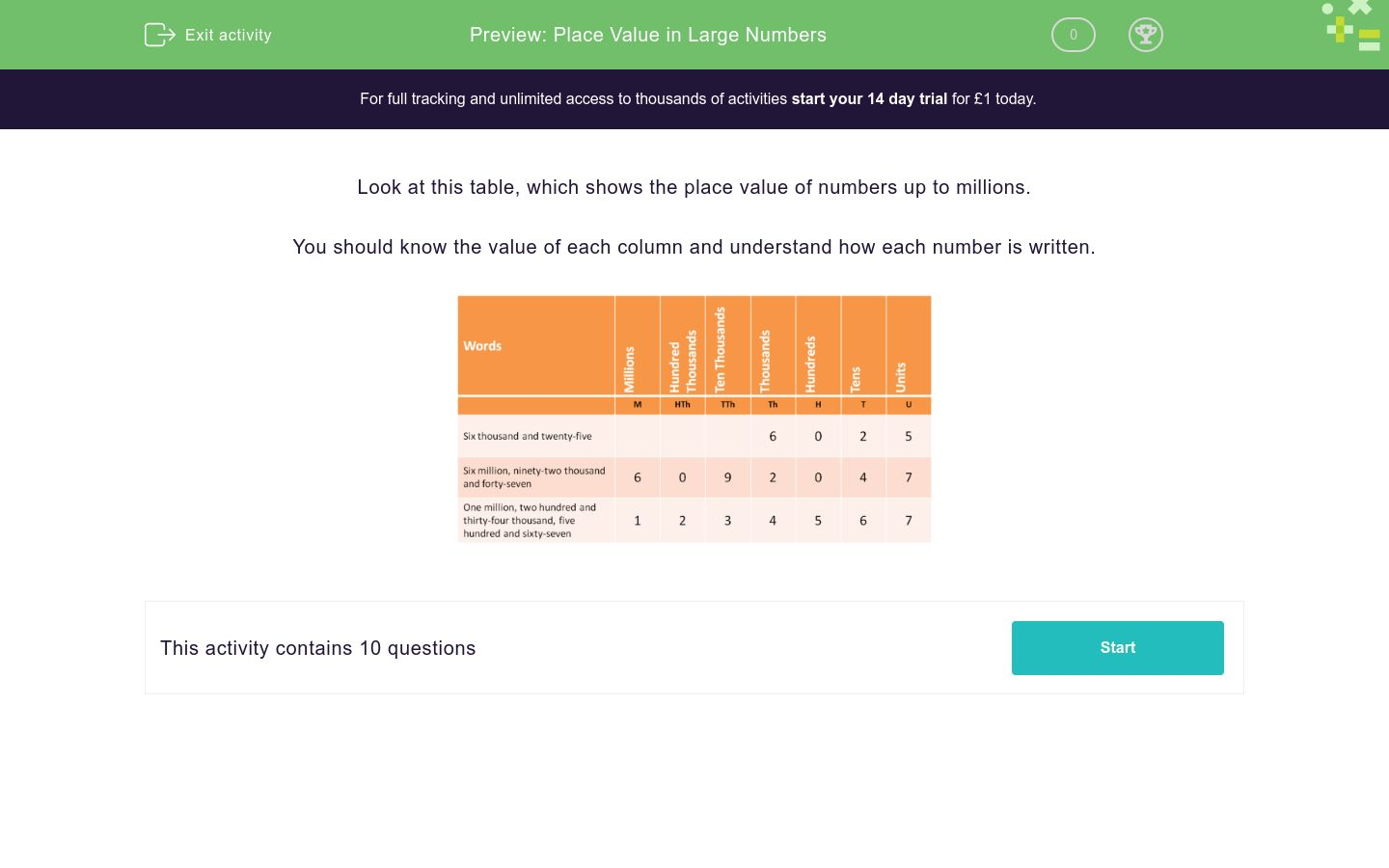# Place Value in Large Numbers

In this worksheet, students increase their fluency of reading numbers up to millions and state the column value of the given digits.Key stage:  KS 3

Curriculum topic:   Number

Curriculum subtopic:   Use Place Value for All Numbers

Difficulty level:### QUESTION 1 of 10

Look at this table, which shows the place value of numbers up to millions.

You should know the value of each column and understand how each number is written.When you write the number 43124, select the column in which you would place the digit  3.

Hundreds

Thousands

Ten Thousands

Hundred Thousands

Millions

Tens

Units

When you write the number 38124, select the column in which you would place the digit  3.

Hundreds

Thousands

Ten Thousands

Hundred Thousands

Millions

Tens

Units

When you write the number 308124, select the column in which you would place the digit  3.

Hundreds

Thousands

Ten Thousands

Hundred Thousands

Millions

Tens

Units

When you write the number 5308124, select the column in which you would place the digit  3.

Hundreds

Thousands

Ten Thousands

Hundred Thousands

Millions

Tens

Units

When you write the number 5308124, select the column in which you would place the digit  2.

Hundreds

Thousands

Ten Thousands

Hundred Thousands

Millions

Tens

Units

When you write the number 5308124, select the column in which you would place the digit  5.

Hundreds

Thousands

Ten Thousands

Hundred Thousands

Millions

Tens

Units

When you write the number 5308124, select the column in which you would place the digit  8.

Hundreds

Thousands

Ten Thousands

Hundred Thousands

Millions

Tens

Units

When you write the number 507961, select the column in which you would place the digit  0.

Hundreds

Thousands

Ten Thousands

Hundred Thousands

Millions

Tens

Units

When you write the number 507961, select the column in which you would place the digit  9.

Hundreds

Thousands

Ten Thousands

Hundred Thousands

Millions

Tens

Units

When you write the number 5007001, select the column in which you would place the digit  5.

Hundreds

Thousands

Ten Thousands

Hundred Thousands

Millions

Tens

Units

• Question 1

When you write the number 43124, select the column in which you would place the digit  3.

Thousands
• Question 2

When you write the number 38124, select the column in which you would place the digit  3.

Ten Thousands
• Question 3

When you write the number 308124, select the column in which you would place the digit  3.

Hundred Thousands
• Question 4

When you write the number 5308124, select the column in which you would place the digit  3.

Hundred Thousands
• Question 5

When you write the number 5308124, select the column in which you would place the digit  2.

Tens
• Question 6

When you write the number 5308124, select the column in which you would place the digit  5.

Millions
• Question 7

When you write the number 5308124, select the column in which you would place the digit  8.

Thousands
• Question 8

When you write the number 507961, select the column in which you would place the digit  0.

Ten Thousands
• Question 9

When you write the number 507961, select the column in which you would place the digit  9.

Hundreds
• Question 10

When you write the number 5007001, select the column in which you would place the digit  5.